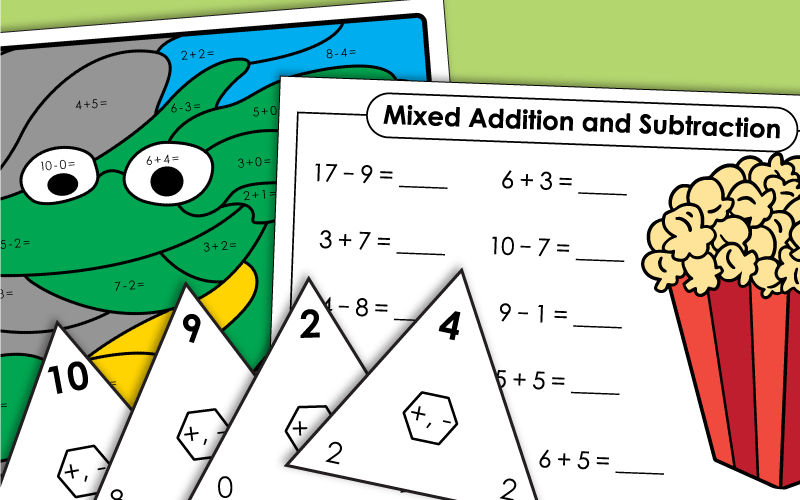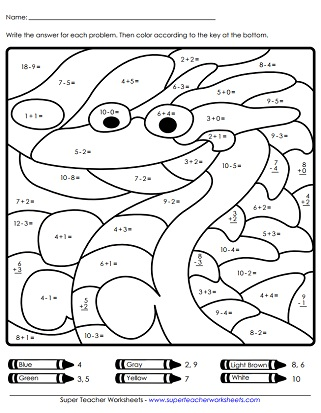# Basic Addition and Subtraction Mix

These printable math worksheets have a mix of both basic addition and basic subtraction problems.## Number Lines

This page has number lines that show an addition or subtraction problem. Write the correct math fact for each.

## Practice Worksheets

Practice addition and subtraction facts with this worksheet. All numbers are single-digit.
In this practice worksheet students will need to seamlessly switch between addition and subtraction. Facts range from 0-20.
Solve all of the mixed addition and subtraction facts as quickly as possible. Includes sums up to and including 20 and differences up to and including 10.
This printable worksheet features twenty basic horizontal addition and subtraction problems.
This printable math worksheet is another version of mixed addition and subtraction math facts laid out horizontally.
Students will write the answers to the addition and subtraction facts on the caterpillar. Addends and minuends are all 10 or less.
Print this worksheet to have your class practice their addition and subtraction facts. Minuends and addends are all 20 or less.

## Mystery Pictures

Add and subtract to find the answers to the math problems. Then color each section of the dragon picture according to the key near the bottom of the worksheet.

## Word Problems

This worksheet focuses on basic addition and subtraction. The numbers in each problem are single-digit.
These word problems tests students knowledge of addition and subtraction facts up to 20.

## Flashcards

These triangle-shaped flashcards can be used to review addition or subtraction facts.

## Speed Math

This worksheet has 50 different addition and subtraction basic facts. Use it as a timed review or quiz.

## Basic Facts

Some of the basic facts are written correctly, but some are not. For facts with incorrect answers, students cross out the sum or difference and replace it with the correct answer.
Tell whether each addition or subtraction statement is true or false? This activity focuses on the meaning of the equal sign in an equation.

STW has scores of worksheets for teaching basic addition skills. Print addition mystery pictures, flashcards, and addition card games.

Basic Subtraction Worksheets

This page has a large collection of basic subtraction worksheets. Download and print a subtraction dice game, bingo activity, math mystery pictures, and number line activities.

## Pictures of Our WorksheetsMy Account
Site Information